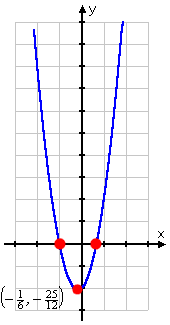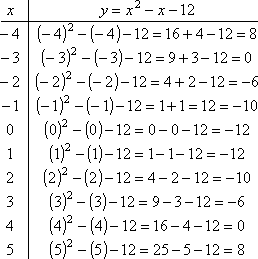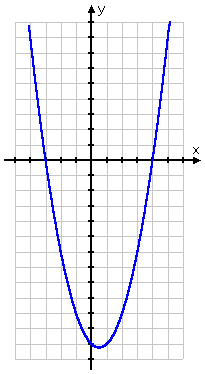Graphing Quadratic Functions: Examples (page 3 of 4)

Sections: Introduction, The meaning of the leading coefficient / The vertex, Examples• Find the vertex and intercepts of y = 3x2 + x – 2 and graph; remember to label the vertex and the axis of symmetry.

This is the same quadratic as in the last example. I already found the vertex when I worked the problem above. This time, I also need to find the intercepts before I do my graph. To find the y-intercept, I set x equal to zero and solve:

y = 3(0)2 + (0) – 2
= 0 + 0 – 2 = –2

Then the y-intercept is the point (0, –2). To find the x-intercept, I set y equal to zero, and solve:

0 = 3x2 + x – 2
0 = (3x – 2)(x + 1)
3x – 2 = 0  or   x + 1 = 0
x2/3   or  x = – 1

Then the x-intercepts are at the points (–1, 0) and ( 2/3, 0).

 The axis of symmetry is halfway between the two x-intercepts at (–1, 0) and at ( 2/3 , 0); using this, I can confirm the answer from the previous page: (–1 + 2/3) / 2 = (–1/3) / 2 = –1/6 The complete answer is a listing of the vertex, the axis of symmetry, and all three intercepts, along with a nice neat graph:     The vertex is at ( –1/6 , –25/12 ), the axis of symmetry is the line x = –1/6 , and the intercepts are at (0, –2), (–1, 0), and ( 2/3, 0).• Find the intercepts, the axis of symmetry, and vertex of y = x2x – 12.

To find the y-intercept, I set x equal to 0 and solve:

y = (0)2 – (0) – 12 = 0 – 0 – 12 = –12

To find the x-intercept, I set y equal to 0 and solve:

0 = x2x – 12

0 = (x – 4)(x + 3)

x = 4  or  x = –3

To find the vertex, I look at the coefficients: a = 1 and b = –1. Plugging into the formula, I get:

h = –(–1)/2(1) = 1/2 = 0.5

To find k, I plug h = 1/2 in for x inside y = x2x – 12, and simplify:

k = (1/2)2 – (1/2) – 12 = 1/41/2 – 12 =  –12.25

Once I have the vertex, it's easy to write down the axis of symmetry: x = 0.5. Now I'll find some additional plot points, to fill in the graph:For my own convenience, I picked x-values that were centered around the x-coordinate of the vertex. Now I can plot the parabola:   Copyright © Elizabeth Stapel 2002-2011 All Rights ReservedThe vertex is at the point (0.5, –12.25),
the axis of symmetry is the line
x = 0.5,

and the intercepts are at the points (0, –12)(–3, 0), and (4, 0).

<< Previous  Top  |  1 | 2 | 3 | 4  |  Return to Index  Next >>